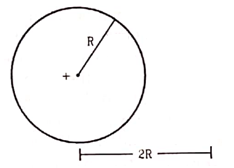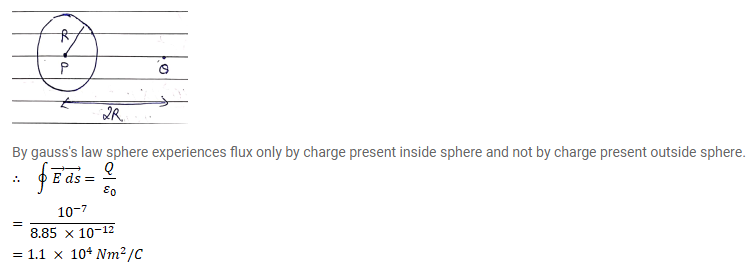# Find the flux of the electric field through a spherical surface ofQuestion:

Find the flux of the electric field through a spherical surface of radius $\mathrm{R}$ due to a charge of $10^{-7} \mathrm{C}$ at the centre and another equal charge at a point $2 R$ away from the centre.Solution: